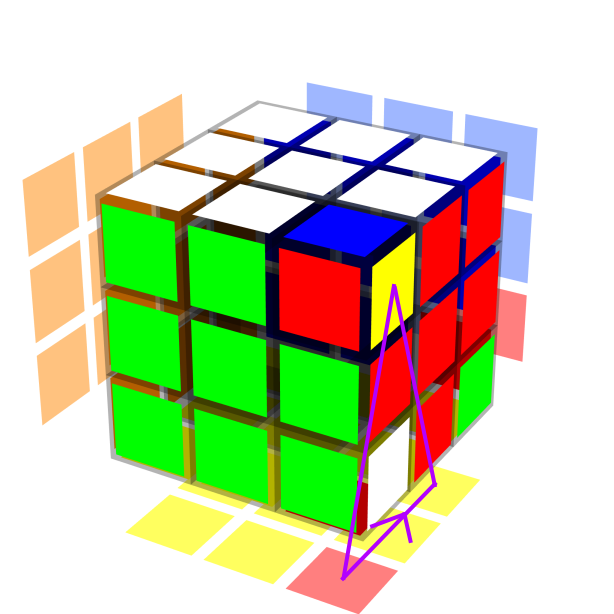## Reconceptualizing the Niklas Commutator

Written April 15, 2022.

The Niklas commutator isn’t as special as I thought. I used to see it as a black box algorithm, but now I see it as a more intuitive commutator.

Note: The end of this article contains demos of several of the algorithms I mention below.

### Backstory

When I first learned to solve the 3x3x3, I learned about the algorithm U R U’ L’ U R’ U’ L. The algorithm permutes three corners on the top layer, though one can see it as orienting three corners on the top layer as well.

Then, I learned about commutators. Commutators are algorithms of the form [X, Y] = X Y X’ Y’, where X and Y themselves can be algorithms, and X’ (pronounced “X prime”) means to do the inverse of X (cubers usually use X’ rather than X-1 or inv(X) to denote “the inverse of X”). Cubers (aka twisty puzzle solvers) can use commutators to move around pieces in a more controlled manner. In other words, they can move pieces to where they want them to go, without messing up other pieces in the process.

When I first learned about commutators, I relied heavily on the commutator [R U R’, D’], or R U R’ D’ R U’ R’ D in full. I knew this commutator moved the RFU sticker to DFR, the DFR sticker to DRB, and the DRB sticker back to RFU. I relied on that sticker movement pattern (Figure 1) to understand how the commutator worked.Figure 1: R U R’ D’ R U’ R’ D applied to the solved state. The RFU->DFR->DRB sticker movement pattern is shown in purple.

I saw the [R U R’, D’] commutator as very different from the Niklas commutator, which I learned as [R, U’ L’ U]. It turns out the Niklas commutator I learned is the mirror inverse of U R U’ L’ U R’ U’ L. That is, the inverse of [R, U’ L’ U] is [U’ L’ U, R], and the mirror of [U’ L’ U, R] along the left-right (L-R) axis is [U R U’, L’], or U R U’ L’ U R’ U’ L in full.

### Equivalent algorithms

One can relate an algorithm to its inverse, mirrors, and conjugations by rotations. To formalize this concept, we can talk about two algorithms being equivalent, along with the concept of equivalence classes.

An algorithm C is equivalent to an algorithm D (we denote this by C ~ D) if and only if at least one of the following holds:

• C is a mirror of D (i.e. C is the same as D but reflected along a plane cutting through the middle of the puzzle)
• C is an inverse of D (i.e. C = D’)
• C is a conjugation by rotation of D (e.g. if C = [z: D] = z D z’)
• C is a composition of the operations above on D (e.g. if C is the mirror inverse of D)

We then call ~ an equivalence relation because it satisfies the following properties:

• Reflexivity: A ~ A for any algorithm A.
• Symmetry: If A ~ B, then B ~ A for any algorithms A and B.
• Transitivity: If A ~ B and B ~ C, then A ~ C for any algorithms A, B, and C.

Then, given a set of algorithms that are equivalent under the equivalence relation ~, we can say that those algorithms belong to the same equivalence class. It also turns out that we can replace the word “algorithm” with “commutator” for the definitions above, and produce a valid definition for the equivalence of two commutators, as well as an equivalence relation between commutators.

### The realization

When I learned about commutators, I already saw [R, U’ L’ U] and [U’ L’ U, R] as being in the same equivalence class (they are inverses of each other). But, I didn’t see [R, U’ L’ U] and [R U R’, D’] as being in the same equivalence class. Additionally, whereas I understood [R U R’, D’] intuitively, I treated [R, U’ L’ U] like a black box.

Yet, the two commutators are actually equivalent by the equivalence relation above. In particular, the inverse of [R, U’ L’ U] is [U’ L’ U, R]. Then, [z’: [U’ L’ U, R]] = [R’ U’ R, D]. Reflecting that commutator along the F-B (front-back) axis gives [R U R’, D’], so [R, U’ L’ U] is equivalent to [R U R’, D’] by the equivalence relation ~ above (Figure 2).Figure 2: [R U R’, D’] and [R, U’ L’ U] side by side. The two commutators are equivalent.Left: [R U R’, D’] applied to the solved state. The RFU->DFR->DRB sticker movement pattern is shown in purple.Right: [R, U’ L’ U] applied to the solved state. The ULB->RFU->RUB sticker movement pattern is shown in purple.

Knowing that the two commutators are equivalent is helpful. For example, I now see [R, U’ L’ U] in a much more intuitive way, and can use it more often while solving the 3x3x3. Another example is that I knew about [R U2 R’, D2] for a long time, but I never thought to use [R2, U’ L2 U], an equivalent commutator, until recently. It also turns out [D2, R’ U2 R] = [z’: [R2, U’ L2 U]] is yet another equivalent commutator, which gave me a way to solve the 3x3x2 edges-first from a different angle.

### Demos

A site that one can use to showcase algorithms and commutators is Twizzle.

Here are some links demonstrating algorithms in the same equivalence class as [R, U’ L’ U]:

Here are some links demonstrating algorithms in the same equivalence class as [R2, U’ L2 U]:

Also, I do not work on or contribute to Twizzle. I’m simply an end user.

Go back to Articles